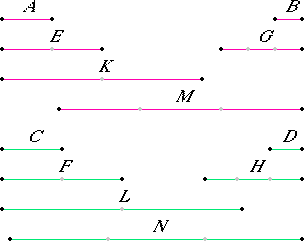# Proposition 4

If a first magnitude has to a second the same ratio as a third to a fourth, then any equimultiples whatever of the first and third also have the same ratio to any equimultiples whatever of the second and fourth respectively, taken in corresponding order.

Let a first magnitude A have to a second B the same ratio as a third C to a fourth D, and let equimultiples E and F be taken of A and C, and G and H other, arbitrary, equimultiples of B and D.

I say that E is to G as F is to H.Take equimultiples K and L of E and F, and other, arbitrary, equimultiples M and N of G and H.

V.3

Since E is the same multiple of A that F is of C, and equimultiples K and L of E and F have been taken, therefore K is the same multiple of A that L is of C. For the same reason M is the same multiple of B that N is of D.

V.Def.5

And, since A is to B as C is to D, and equimultiples K and L have been taken of A and C, and other, arbitrary, equimultiples M and N of B and D, therefore, if K is in excess of M, then L is in excess of N; if it is equal, equal; and if less, less.

V.Def.5

And K and L are equimultiples of E and F, and M and N are other, arbitrary, equimultiples of G and H, therefore E is to G as F is to H.

Therefore, if a first magnitude has to a second the same ratio as a third to a fourth, then any equimultiples whatever of the first and third also have the same ratio to any equimultiples whatever of the second and fourth respectively, taken in corresponding order.

Q.E.D.

## Guide

The statement of the proposition says that

if a : b = c : d, then pa : qb = pc : qd for any numbers p and q.

Note how Euclid uses the definition to prove that the two ratios pa : qb and pc : qd are the same. (Here, a and b are magnitudes of one kind, and c and d are magnitudes of another kind, but p and q are numbers.) We are given a : b = c : d. That means for any numbers m and n that

if ma >=< nb, then mc >=< nd.

We have to prove that pa : qb = pc : qd for any numbers p and q. That means, we have to prove that for any m and n,

if mpa >=< nqb, then mpc >=< nqd.

But that’s just a special case of the given relation

if ma >=< nb, then mc >=< nd.

#### Use of this proposition

Proposition V.4 is used in the proof of one other proposition, namely, V.22.